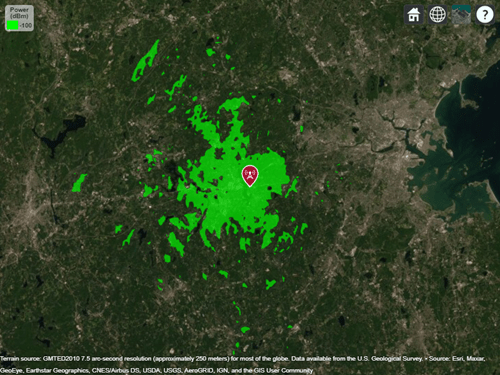# TIREM

TIREM propagation model

## Description

Model the behavior of electromagnetic radiation from a point of transmission as it travels over irregular terrain, including buildings, by using the Terrain Integrated Rough Earth Model™ (TIREM™) model. Represent the TIREM model by using a `TIREM` object.

The TIREM model:

• Is valid from 1 MHz to 1000 GHz.

• Calculates path loss from free-space loss, terrain and obstacle diffraction, ground reflection, atmospheric refraction, and tropospheric scatter.

• Provides path loss estimates by combining physics with empirical data.

`TIREM` objects require access to an external TIREM library. For more information, see Access TIREM Software.

## Creation

Create a `TIREM` object by using the `propagationModel` function.

## Properties

expand all

Polarization of transmitter and receiver antennas, specified as `"horizontal"` or `"vertical"`. The object assumes both antennas have the same polarization. The model uses this value to calculate path loss due to ground reflection.

Data Types: `char` | `string`

Conductivity of the ground, specified as a numeric scalar in siemens per meter (S/m) in the range [0.0005, 100]. The model uses this value to calculate path loss due to ground reflection. The default value corresponds to average ground.

Data Types: `double`

Relative permittivity of the ground, specified as a numeric scalar in the range [1, 100]. Relative permittivity is expressed as a ratio of absolute material permittivity to the permittivity of vacuum. The model uses this value to calculate the path loss due to ground reflection. The default value corresponds to average ground.

Data Types: `double`

Atmospheric refractivity near the ground, specified as a numeric scalar in N-units in the range [250, 400]. The model uses this value to calculate the path loss due to refraction through the atmosphere and tropospheric scatter. The default value corresponds to average atmospheric conditions.

Data Types: `double`

Absolute air humidity near the ground, specified as a numeric scalar in grams per cubic meter (`g/m3`) in the range [0, 110]. You can use this value to calculate path loss due to atmospheric absorption. The default value corresponds to the absolute humidity of air at 15 degrees Celsius and 70 percent relative humidity.

Data Types: `double`

## Object Functions

 `pathloss` Path loss of radio wave propagation `add` Add propagation models

## Examples

collapse all

Display the coverage area for a transmitter using the TIREM model.

```pm = propagationModel("tirem"); tx = txsite("Name","Apple Hill","Latitude",42.3001,"Longitude",-71.3604); coverage(tx,pm)```